Next: Numerical simulation Up: Examples Previous: Numerical simulation

## Unpaired Group Difference

We assume that the individual subjects are grouped in two groups and that within each group the first-level parameters are normally distributed around a group-specific mean. That is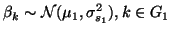andIn order to simplify further notation and without loss of generality we assume that the subjects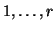belong to the first group and subjects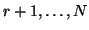belong to the second group. We do not make any assumption about the first-level covariance structure and simply set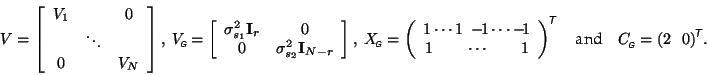Then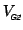is block diagonal with elements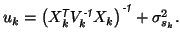If we define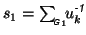and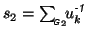, the group parameter estimate writes as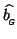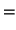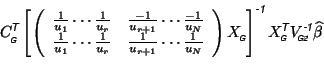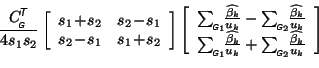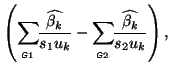where the variance, as usual, is calculated from the first term as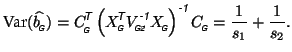Under the same assumptions as before, of equal covariance at the first level and normalised designs (i.e. homoscedastic model), these equation simplify to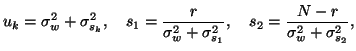and thuswith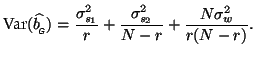Note that the second level contrast includes an appropriate scaling constant. This factor becomes irrelevant once the group parameter of interest is combined with its variance to form a test statistic.

SubsectionsNext: Numerical simulation Up: Examples Previous: Numerical simulation
Christian Beckmann 2003-07-16